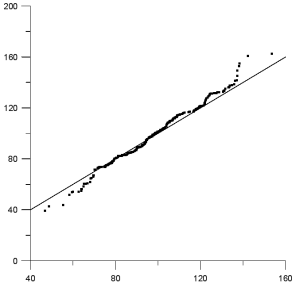# Q-Q Plots and Normal Q-Q Plots

 Click the Home | New Graph | Statistical | Q-Q Plot or the Home | New Graph | Statistical | Normal Q-Q Plot command to plot a Q-Q plot. A Q-Q plot is a type of probability plot which compares the probability distribution of two data sets by plotting their quantiles against each other. Each point plotted on the Q-Q plot represents the same quantile in each data set. If the two distributions being compared have similar distributions, the points will approximately lie on the line y=x. If the distributions are linearly related, the points will lie on a line, but not necessarily the y=x line. Click the Q-Q Plot or Normal Q-Q Plot buttons to create the desired plot type.The Normal Q-Q Plot plots the distribution of a single data set against the normal distribution. To change a Q-Q plot to a Normal Q-Q plot, change either the X variable or Y variable property on the Property Manager Plot page to None.

The y=x line is also plotted on both the Q-Q plot and the normal Q-Q plot.Q-Q plots show data as symbols with a y=x line This plot compares two data sets that are closely related, so the data fall almost always along the y=x line.

## Creating a Q-Q Plot or Normal Q-Q Plot

To create a new Q-Q plot or normal Q-Q plot:

1. Click the Home | New Graph | Statistical | Q-Q Plot or the Home | New Graph | Statistical | Normal Q-Q Plot command.
2. Select a data file in the Open Worksheet dialog. You can select a new data file or you can select an open data file in the Open worksheets section.
3. Click the Open button. A Q-Q plot or normal Q-Q plot is created using the default properties.

## Editing a Q-Q Plot or Normal Q-Q Plot

To change the features of a Q-Q plot or a normal Q-Q plot, click once on the plot in either the plot window or the Object Manager to select it. The properties of the selected Q-Q plot are displayed in the Property Manager.

Click the following tabs in the Property Manager to change different properties:

## Graph Properties

The graph properties contain the following pages in the Property Manager.